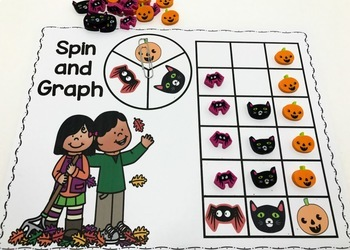# Halloween Mini Eraser Math ActivitiesSubject
Resource Type
File Type

PDF

(3 MB|40 pages)
Standards
• Product Description
• StandardsNEW

Fun math activities to go along with the jack o'lantern, black cat, skulls and spider mini erasers available at Target in 2017! Your students will love practicing number recognition, one to one correspondence and patterning with these fun centers!

Contents:
4 ten frame mats - two for number 0-10 and two for 0-20.
0-20 number card sets for the black cat, spider,skull and jack o'lantern erasers.
Spider web cards from 0 - 10 to use with the spider erasers.
2 mats for patterning with the spider webs - features AB, AABB, AAB and ABC patterns.
2 What Makes Ten mats for the spiders and skulls to practice combinations of ten.
2 Roll & Cover mats - one for number recognition with one dice and one for basic addition with 2 dice.
1 Spin & Graph Mat
1 Roll & Graph dice and graph mat
0-10 ten frame cards for all 4 types of erasers.

Related Products

Add and subtract within 20, demonstrating fluency for addition and subtraction within 10. Use strategies such as counting on; making ten (e.g., 8 + 6 = 8 + 2 + 4 = 10 + 4 = 14); decomposing a number leading to a ten (e.g., 13 - 4 = 13 - 3 - 1 = 10 - 1 = 9); using the relationship between addition and subtraction (e.g., knowing that 8 + 4 = 12, one knows 12 - 8 = 4); and creating equivalent but easier or known sums (e.g., adding 6 + 7 by creating the known equivalent 6 + 6 + 1 = 12 + 1 = 13).
Fluently add and subtract within 5.
For any number from 1 to 9, find the number that makes 10 when added to the given number, e.g., by using objects or drawings, and record the answer with a drawing or equation.
Organize, represent, and interpret data with up to three categories; ask and answer questions about the total number of data points, how many in each category, and how many more or less are in one category than in another.
Identify whether the number of objects in one group is greater than, less than, or equal to the number of objects in another group, e.g., by using matching and counting strategies.
Total Pages
40 pages
N/A
Teaching Duration
N/A
Report this Resource to TpT
Reported resources will be reviewed by our team. Report this resource to let us know if this resource violates TpT’s content guidelines.